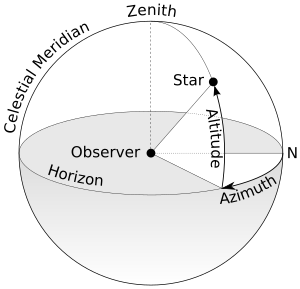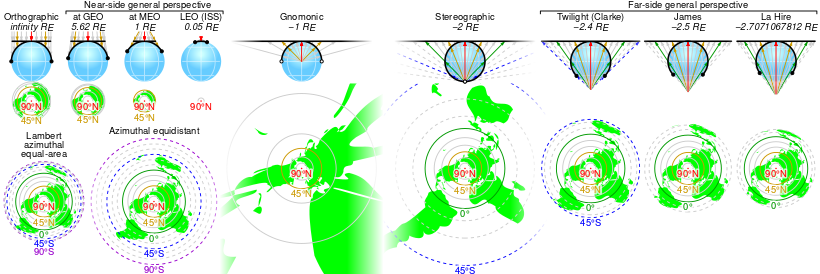# السمت

السمت إنگليزية: Azimuth هو زاوية بين مستوي مرجعي ونقطة، غالباً ما يقاس بالدرجة. يستخدم السمت في العديد من العلوم التطبيقية مثل الملاحة، الفلك وعلم المساحة، وفي سلاح المدفعية. كلمة سمت العربية هي أصل كلمة Azimuth الإنكليزية، وهي في الأساس تعني الطريق، وكانت تشير إلى وجه السفن أو الأشخاص أثناء الترحال قديماً.The azimuth is the angle formed between a reference direction (in this example north) and a line from the observer to a point of interest projected on the same plane as the reference direction orthogonal to the zenith.

السمت هي النقطة التي تكون في الكرة السماوية فوق رأس الراصد تماما ، والبعد السمتي للنجم هو البعد الزاوي من السمت إلى النجـــــم.

Mathematically, the relative position vector from an observer (origin) to a point of interest is projected perpendicularly onto a reference plane (the horizontal plane); the angle between the projected vector and a reference vector on the reference plane is called the azimuth.

When used as a celestial coordinate, the azimuth is the horizontal direction of a star or other astronomical object in the sky. The star is the point of interest, the reference plane is the local area (e.g. a circular area with a 5 km radius at sea level) around an observer on Earth's surface, and the reference vector points to true north. The azimuth is the angle between the north vector and the star's vector on the horizontal plane.

Azimuth is usually measured in degrees (°). The concept is used in navigation, astronomy, engineering, mapping, mining, and ballistics.

. . . . . . . . . . . . . . . . . . . . . . . . . . . . . . . . . . . . . . . . . . . . . . . . . . . . . . . . . . . . . . . . . . . . . . . . . . . . . . . . . . . . . . . . . . . . . . . . . . . . . . . . . . . . . . . . . . . . . . . . . . . . . . . . . . . . . . . . . . . . . . . . . . . . . . . . . . . . . . . . . . . . . . . .

## في الفلك

In the horizontal coordinate system, used in celestial navigation, azimuth is one of the two coordinates. The other is altitude, sometimes called elevation above the horizon. It is also used for satellite dish installation (see also: sat finder). In modern astronomy azimuth is nearly always measured from the north.

## في الملاحة

In land navigation, azimuth is usually denoted alpha, α, and defined as a horizontal angle measured clockwise from a north base line or meridian. Azimuth has also been more generally defined as a horizontal angle measured clockwise from any fixed reference plane or easily established base direction line.

Today, the reference plane for an azimuth is typically true north, measured as a 0° azimuth, though other angular units (grad, mil) can be used. Moving clockwise on a 360 degree circle, east has azimuth 90°, south 180°, and west 270°. There are exceptions: some navigation systems use south as the reference vector. Any direction can be the reference vector, as long as it is clearly defined.

Quite commonly, azimuths or compass bearings are stated in a system in which either north or south can be the zero, and the angle may be measured clockwise or anticlockwise from the zero. For example, a bearing might be described as "(from) south, (turn) thirty degrees (toward the) east" (the words in brackets are usually omitted), abbreviated "S30°E", which is the bearing 30 degrees in the eastward direction from south, i.e. the bearing 150 degrees clockwise from north. The reference direction, stated first, is always north or south, and the turning direction, stated last, is east or west. The directions are chosen so that the angle, stated between them, is positive, between zero and 90 degrees. If the bearing happens to be exactly in the direction of one of the cardinal points, a different notation, e.g. "due east", is used instead.

### True north-based azimuths

From north, eastern side
Direction Azimuth
North
North-northeast 22.5°
Northeast 45°
East-northeast 67.5°
East 90°
East-southeast 112.5°
Southeast 135°
South-southeast 157.5°
From north, western side
Direction Azimuth
South 180°
South-southwest 202.5°
Southwest 225°
West-southwest 247.5°
West 270°
West-northwest 292.5°
Northwest 315°
North-northwest 337.5°

## In geodesy

We are standing at latitude $\varphi _{1}$ , longitude zero; we want to find the azimuth from our viewpoint to Point 2 at latitude $\varphi _{2}$ , longitude L (positive eastward). We can get a fair approximation by assuming the Earth is a sphere, in which case the azimuth α is given by

$\tan \alpha ={\frac {\sin L}{\cos \varphi _{1}\tan \varphi _{2}-\sin \varphi _{1}\cos L}}$

A better approximation assumes the Earth is a slightly-squashed sphere (an oblate spheroid); azimuth then has at least two very slightly different meanings. Normal-section azimuth is the angle measured at our viewpoint by a theodolite whose axis is perpendicular to the surface of the spheroid; geodetic azimuth (or geodesic azimuth) is the angle between north and the ellipsoidal geodesic (the shortest path on the surface of the spheroid from our viewpoint to Point 2). The difference is usually negligible: less than 0.03 arc second for distances less than 100 km.

Normal-section azimuth can be calculated as follows:[بحاجة لمصدر]

{\begin{aligned}e^{2}&=f(2-f)\\1-e^{2}&=(1-f)^{2}\\\Lambda &=\left(1-e^{2}\right){\frac {\tan \varphi _{2}}{\tan \varphi _{1}}}+e^{2}{\sqrt {\frac {1+\left(1-e^{2}\right)\left(\tan \varphi _{2}\right)^{2}}{1+\left(1-e^{2}\right)\left(\tan \varphi _{1}\right)^{2}}}}\\\tan \alpha &={\frac {\sin L}{(\Lambda -\cos L)\sin \varphi _{1}}}\end{aligned}}

where f is the flattening and e the eccentricity for the chosen spheroid (e.g., 1298.257223563 for WGS84). If φ1 = 0 then

$\tan \alpha ={\frac {\sin L}{\left(1-e^{2}\right)\tan \varphi _{2}}}$

To calculate the azimuth of the sun or a star given its declination and hour angle at our location, we modify the formula for a spherical earth. Replace φ2 with declination and longitude difference with hour angle, and change the sign (since the hour angle is positive westward instead of east).[بحاجة لمصدر]

## في رسم الخرائط

A standard Brunton Geo compass, commonly used by geologists and surveyors to measure azimuth

The cartographical azimuth or grid azimuth (in decimal degrees) can be calculated when the coordinates of 2 points are known in a flat plane (cartographical coordinates):

$\alpha ={\frac {180}{\pi }}\operatorname {atan2} (X_{2}-X_{1},Y_{2}-Y_{1})$

Remark that the reference axes are swapped relative to the (counterclockwise) mathematical polar coordinate system and that the azimuth is clockwise relative to the north. This is the reason why the X and Y axis in the above formula are swapped. If the azimuth becomes negative, one can always add 360°.

The formula in radians would be slightly easier:

$\alpha =\operatorname {atan2} (X_{2}-X_{1},Y_{2}-Y_{1})$

Note the swapped $(x,y)$  in contrast to the normal $(y,x)$  atan2 input order.

The opposite problem occurs when the coordinates (X1, Y1) of one point, the distance D, and the azimuth α to another point (X2, Y2) are known, one can calculate its coordinates:

{\begin{aligned}X_{2}&=X_{1}+D\sin \alpha \\Y_{2}&=Y_{1}+D\cos \alpha \end{aligned}}

This is typically used in triangulation and azimuth identification (AzID), especially in radar applications.

### مساقط الخرائط

There is a wide variety of azimuthal map projections. They all have the property that directions (the azimuths) from a central point are preserved. Some navigation systems use south as the reference plane. However, any direction can serve as the plane of reference, as long as it is clearly defined for everyone using that system.مقارنة وبعض الإسقاطات السمتية المتمركزة على 90° ش بنفس المقياس، مرتبة حسب ارتفاع الإسقاط بمضاعفات نصف قطر الأرض. (انقر لمزيد من التفاصيل)

## Related coordinates

### Right ascension

If, instead of measuring from and along the horizon, the angles are measured from and along the celestial equator, the angles are called right ascension if referenced to the Vernal Equinox, or hour angle if referenced to the celestial meridian.

### Polar coordinate

In mathematics, the azimuth angle of a point in cylindrical coordinates or spherical coordinates is the anticlockwise angle between the positive x-axis and the projection of the vector onto the xy-plane. The angle is the same as an angle in polar coordinates of the component of the vector in the xy-plane and is normally measured in radians rather than degrees. As well as measuring the angle differently, in mathematical applications theta, θ, is very often used to represent the azimuth rather than the representation of symbol phi φ.

. . . . . . . . . . . . . . . . . . . . . . . . . . . . . . . . . . . . . . . . . . . . . . . . . . . . . . . . . . . . . . . . . . . . . . . . . . . . . . . . . . . . . . . . . . . . . . . . . . . . . . . . . . . . . . . . . . . . . . . . . . . . . . . . . . . . . . . . . . . . . . . . . . . . . . . . . . . . . . . . . . . . . . . .

## Other uses

For magnetic tape drives, azimuth refers to the angle between the tape head(s) and tape.

In sound localization experiments and literature, the azimuth refers to the angle the sound source makes compared to the imaginary straight line that is drawn from within the head through the area between the eyes.

An azimuth thruster in shipbuilding is a propeller that can be rotated horizontally.

## المراجع

1. ^ قالب:Cite Dictionary.com
2. ^ Rutstrum, Carl, The Wilderness Route Finder, University of Minnesota Press (2000), ISBN 0-8166-3661-3, p. 194
3. ^ U.S. Army, Map Reading and Land Navigation, FM 21-26, Headquarters, Dept. of the Army, Washington, D.C. (7 May 1993), ch. 6, p. 2
4. ^ U.S. Army, Map Reading and Land Navigation, FM 21-26, Headquarters, Dept. of the Army, Washington, D.C. (28 March 1956), ch. 3, p. 63
5. ^ U.S. Army, ch. 6 p. 2
6. ^ U.S. Army, Advanced Map and Aerial Photograph Reading, Headquarters, War Department, Washington, D.C. (17 September 1941), pp. 24–25
7. ^ U.S. Army, Advanced Map and Aerial Photograph Reading, Headquarters, War Department, Washington, D.C. (23 December 1944), p. 15
8. ^ Torge & Müller (2012) Geodesy, De Gruyter, eq.6.70, p.248

## وصلات خارجية

•   Chisholm, Hugh, ed. (1911). "Azimuth" . دائرة المعارف البريطانية (eleventh ed.). Cambridge University Press. Cite has empty unknown parameter: |coauthors= (help); More than one of |encyclopedia= and |encyclopedia= specified (help)
•   "[[s:Collier's New Encyclopedia (1921)/{{{1}}}|{{{1}}}]]". Collier's New Encyclopedia. 1921.